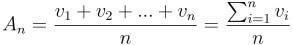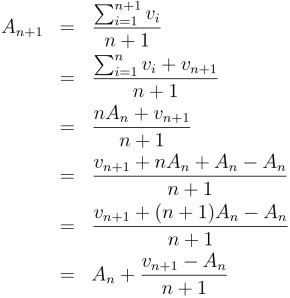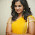2010/01/11

Incremental average calculation

To calculate the average of some numbers, we can use the following simple formula:It works in most cases, but let's suppose that we would like to know the average of a fast growing set of numbers.

Using the formula above can be problematic:
• When a new number is added to the data set, we have to sum all numbers to calculate the current average. As the data set grows large, it takes more and more time to evaluate the formula above.
• The algorithm based on this formula is numerically unstable: the sum of the numbers may overflow, even if the resulting average would not (see the unit test testNumericalStability).

Fortunately we can easily transform the formula to an "incremental" form:This new form is almost as easy to implement as the original, but has two important advantages:
• It can be used to calculate the average incrementally (the current average is calculated from the current value and the previous average).
• It is numerically stable (see the unit test testNumericalStability).

Resources:

1.It's very useful. Thanks you!!

2.3.Thanks. Rewrite equation easy to understand :)

4.Thanks so very much for taking your time to create this very useful and informative site. I have learned a lot from your site. Thanks!!

JAVA Training Institutes in Chennai

5.*the current average is calculated from the current value and the previous average... AND the index of the new element!

Which is also the downside of the algorithm, as we need to retain this information along with the previous average value.

6.Why can't we use the formula where A(n+1) = ((A(n)*(N)+V(n+1))/(N+1). Isn't it the same thing? I also want to the difference. Please explain this to me.